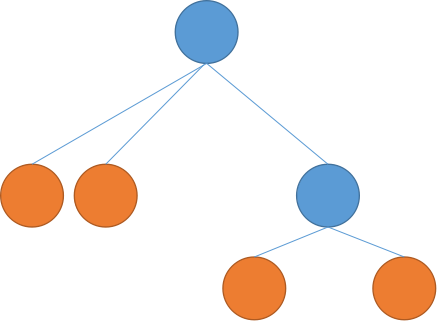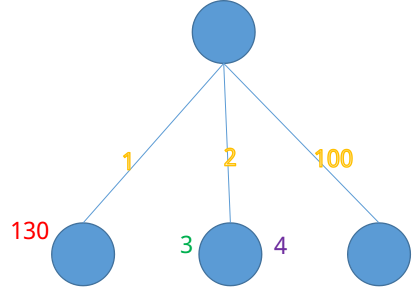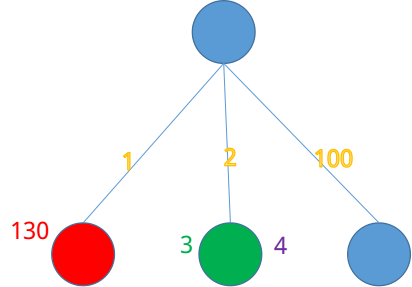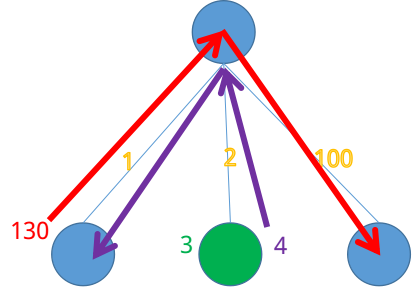# Luogu 1084 NOIP2012 疫情控制 （二分，贪心，倍增）

### Description

H 国有 n 个城市，这 n 个城市用 n-1 条双向道路相互连通构成一棵树， 1 号城市是首都， 也是树中的根节点。
H 国的首都爆发了一种危害性极高的传染病。当局为了控制疫情，不让疫情扩散到边境 城市（叶子节点所表示的城市），决定动用军队在一些城市建立检查点，使得从首都到边境 城市的每一条路径上都至少有一个检查点，边境城市也可以建立检查点。但特别要注意的是， 首都是不能建立检查点的。

4
1 2 1
1 3 2
3 4 3
2
2 2

3

## 解决思路## 代码

#include<iostream>
#include<cstdio>
#include<cstdlib>
#include<cstring>
#include<algorithm>
#include<vector>
using namespace std;

#define ll long long
#define mem(Arr,x) memset(Arr,x,sizeof(Arr))

const int maxN=100001;
const int maxTwo=20;
const ll inf=1e15;

class Edge//边，前向星
{
public:
ll u,v,w;
};

class sort_data//用来排序的，这是在check中记录能到达根节点的点
{
public:
ll pos,resttime;//来源（第一层的点），剩余时间
};

bool operator < (sort_data A,sort_data B)//因为要排序，所以重载小于运算符
{
return A.resttime<B.resttime;
}

class Destination//记录还没有控制的第一层的点，同时记录它与1的距离
{
public:
ll pos,tim;//点编号，与1的距离
};

bool operator < (Destination A,Destination B)//重载小于运算
{
return A.tim<B.tim;
}

ll n,m;
int cnt;
ll Ans;//记录答案
Edge E[maxN];//图
int Next[maxN];//图
ll Armypos[maxN];//记录每一个军队的初始所在点
ll Skip[maxN];//倍增数组，Skip[i][j]代表j号点向上跳2^j个点所到的位置
ll Skip_path[maxN];//倍增数组，Skip_path[i][j]代表对应的Skip[i][j]跳的距离
ll Getpos[maxN];//在check中记录每个军队向上跳最浅能跳到的点
bool is_cover[maxN];//在check中记录某个点是否已经被控制

void Skip_dfs(int u,int father);//初始化倍增的信息
bool check(int mid);//二分检查
void check_dfs(int u,int father);//dfs检查，即这个用来处理把那些儿子都已经被控制的点也置为已控制

int main()
{

cnt=0;
for (int i=1;i<n;i++)//读入树边
{
}
//开始构造倍增数组
mem(Skip,-1);
mem(Skip_path,0);
Skip_dfs(1,1);

for (int i=1;i<=maxTwo;i++)
for (int j=1;j<=n;j++)
if (Skip[i-1][j]!=-1)
{
Skip[i][j]=Skip[i-1][Skip[i-1][j]];
Skip_path[i][j]=Skip_path[i-1][j]+Skip_path[i-1][Skip[i-1][j]];
}
//构造完毕

for (int i=1;i<=m;i++)

int l=0,r=300000;//二分
Ans=inf;
do
{
int mid=(l+r)/2;
if (check(mid))
{
Ans=min(Ans,(ll)(mid));
r=mid-1;
}
else
l=mid+1;
}
while (l<=r);
cout<<Ans<<endl;
return 0;
}

{
ll x=0;
char ch=getchar();
while ((ch>'9')||(ch<'0'))
ch=getchar();
while ((ch>='0')&&(ch<='9'))
{
x=x*10+ch-48;
ch=getchar();
}
return x;
}

{
cnt++;
E[cnt].u=u;
E[cnt].v=v;
E[cnt].w=w;

cnt++;
E[cnt].u=v;
E[cnt].v=u;
E[cnt].w=w;
return;
}

void Skip_dfs(int u,int father)//构造倍增初始数据，即Skip[i]和Skip_path[i]
{
{
int v=E[i].v;
if (v==father)
continue;
if (Skip[v]==-1)
{
Skip[v]=u;
Skip_path[v]=E[i].w;
Skip_dfs(v,u);
}
}
return;
}

bool check(int mid)//二分检查
{
mem(is_cover,0);
vector<sort_data> V;//记录能到达1的军队
V.clear();
for (int i=1;i<=m;i++)//让每一个军队都尽量向上跳
{
Getpos[i]=Armypos[i];//初始位置就是军队所在的位置
ll timecnt=0;//记录当前已花的时间
for (int j=maxTwo;j>=0;j--)
if ((Skip[j][Getpos[i]]>1)&&(timecnt+Skip_path[j][Getpos[i]]<=mid))//注意这里>1，
{
timecnt=timecnt+Skip_path[j][Getpos[i]];
Getpos[i]=Skip[j][Getpos[i]];
}
if ((Skip[Getpos[i]]==1)&&(Skip_path[Getpos[i]]+timecnt<mid))//当还能向上跳一次并且跳到1时，记录
{
V.push_back((sort_data){Getpos[i],mid-timecnt-Skip_path[Getpos[i]]});
}
else//否则则停在这里，直接控制这课子树
is_cover[Getpos[i]]=1;
}

check_dfs(1,1);//dfs检查控制

sort(V.begin(),V.end());//对剩余军队按剩余时间升序排序

vector<Destination> D;//记录还没有被控制的第一层节点
D.clear();
{
int v=E[i].v;
if (is_cover[v]==0)
D.push_back((Destination){v,E[i].w});
}
sort(D.begin(),D.end());//排序第一层点

if (D.size()>V.size())//当剩余军队数小于剩余第一层点数时，不管怎么呢调派军队都无法满足，返回不可行
return 0;
int j=0;//记录当前匹配到第几个点
if (D.size()==0)//若所有第一层点都已匹配，返回可行
return 1;
for (int i=0;i<V.size();i++)//i从小到大枚举每一个军队
{
if (is_cover[V[i].pos]==0)//若当前军队的来源还未控制，则让这支军队直接控制其来源，这样更划算
{
is_cover[V[i].pos]=1;
continue;
}
while ((is_cover[D[j].pos]==1)&&(j<D.size()))//因为有上面这种操作，所以先要让j跳到第一个还未匹配的第一层节点
j++;
if (j==D.size())//当j到末尾时，返回可行
return 1;
if (V[i].resttime>=D[j].tim)//判断当前军队是否可以去控制这个点，如果可以，则去控制
{
is_cover[D[j].pos]=1;
j++;
}
if (j==D.size())
return 1;
}
while ((is_cover[D[j].pos]==1)&&(j<D.size()))//最后再让j向后跳一次
j++;
if (j==D.size())//当到末尾时，返回可行
return 1;
return 0;//否则返回不可行
}

void check_dfs(int u,int father)
{
if (is_cover[u]==1)//已经被控制，直接返回
return;
bool is_all=1;//记录是不是所有的儿子都已经控制
bool has_son=0;//记录是否有儿子
{
int v=E[i].v;
if (v==father)
continue;
has_son=1;
check_dfs(v,u);
if (is_cover[v]==0)
is_all=0;
}
if (has_son==0)//当没有儿子时，肯定没有被控制
is_all=0;
if (is_all==1)//若所有儿子都被控制，则当前也标记为被控制
is_cover[u]=1;
return;
}

posted @ 2017-09-27 22:46  SYCstudio  阅读(...)  评论(...编辑  收藏